Volume 64 Supplement 8

# An improved relative intensity model for earthquake forecasts in Japan

## Abstract

In a basic forecast model, where the expected activity in a future period is in proportion to the observed activity in the past, i.e. a relative intensity model, there are important options which can improve the performance of the model: (1) The expected number of earthquake occurrences at places where no earthquakes have been observed in the past, and (2) the extent of the area of the past reference activity, are considered to be two of the most significant factors. However, these issues have not been fully examined in previous studies of Japan. Taking into consideration the forecast of the expected number of earthquakes with a magnitude of 5 or greater in the Japan area, as designated by the Japanese test center of the Collaboratory for the Study of Earthquake Predictability (CSEP), retrospective experiments result in an optimized value of 0.00085 per year per unit cell in which no earthquakes were observed in the previous 43 years. Here, the size of a unit cell is 0.1° by 0.1° in latitude and longitude, with depths from the surface to 100 km. In addition, an area of 0.3° by 0.3° in latitude and longitude was found to be the best spatial extent for the reference activity.

## 1. Introduction

A long-term average of seismic activity has been considered to be useful information regarding the forecasting of future earthquakes, as discussed by many authors. In earlier years, discussions were limited to the spatial variation in activity and/or mean recurrence times (e.g., Mallet, 1858; Sekiya, 1899; Gutenberg and Richter, 1949). Since the 1960s, the discussion has become more realistic by incorporating the Gutenberg-Richter law (e.g., Gutenberg and Richter, 1944) for the frequency-magnitude relation. Based on earthquakes observed during 29 years, for example, Allen et al. (1965) calculated the expected magnitudes of earthquakes that occur once-per-year and once-per-100 years, for 6 sub-regions in southern California. Nishenko and Bollinger (1990) presented probabilities of earthquake occurrence for several regions in the United States in the next 10 to 100 years for magnitude ranges equal to, or greater than, 6.1 and 7.4.

Recently, various forecast models based on long-term activity have been proposed (e.g., Kagan and Jackson, 2000; Kafka, 2002; Rhoades and Evison, 2004; Kossobokov, 2004; Nanjo et al., 2006; Helmstetter et al., 2007; Holliday et al., 2007; Kagan et al., 2007; Zechar and Jordan, 2010). Among these studies, the simplest idea, i.e. a relative intensity (RI) model, is that the expected activity in a future period is proportional to the observed activity in the past, as discussed by Tiampo et al. (2002), Rundle et al. (2002), and Holliday et al. (2005), for example. Also, Nanjo (2011) has applied the relative intensity model to the Japan area.

In the relative intensity model, it is emphasized that the occurrence of large earthquakes can be forecast using the activity of smaller earthquakes, even with a limitation in the available period of data. Although the conversion of magnitude ranges is not discussed in the present paper, the term relative intensity is used to present a simple assumption between activity in the past and the future. The magnitude range can easily be changed, if necessary, with the assumption of a plausible b value in the Gutenberg-Richter law.

In the case of a model where the seismic activity is smoothed with a weighting function that extends over a sufficiently wide area, a certain number of earthquakes may always be expected to occur within the whole area. In any relative intensity, or related, models, however, special caution is needed for unit areas in which no earthquakes have occurred in the past (hereafter, such an area is called a cell of zero activity). If no earthquakes are incorrectly forecast in such an area, the model performance diverges to −∞ in terms of the log-likelihood of the earthquake occurrence during the forecast period. In order to avoid such a situation, a certain earthquake occurrence probability should be assigned to cells of zero activity. The first question is: what is a reasonable value?

Second, it is also important to take into account the space-time extent (i.e., reference area and reference period) over which the past seismicity is smoothed. Since it is difficult to assume that there is no correlation of activity between cells over short distances, a reasonable spatial extent should be examined for each studied region in order to provide a better forecasting performance, as discussed by many authors. A reasonable length of time for the reference period should also be evaluated by the available data.

In order to propose an improved relative intensity model for the Japan area, the issues mentioned above are discussed in the present paper, because they have not been thoroughly investigated in past studies. The study area is all of Japan which is one of the specified regions designated by the Japanese test center (Nanjo et al., 2011; Tsuruoka et al., 2012) of the Collaboratory for the Study of Earthquake Predictability (CSEP; e.g., Jordan, 2006; Schorlemmer et al., 2010; Zechar et al., 2010a). The area is shown in Fig. 1, and consists of 20,062 rectangular cells, each with a size of 0.1° by 0.1° in latitude and longitude, and depths from the surface to 100 km.

Among several test classes of the forecast period for the Japanese CSEP experiment, the 1-year forecast of earthquakes is investigated here based on the earthquake catalog provided by the Japan Meteorological Agency (JMA). Although earthquakes in 1923 and later are listed in the JMA catalog, the data between 1965 and 2008 are mainly used here, considering the reliability of the catalog (e.g., Ishikawa, 1987) and the fact that the recent data of 2009–2010 has not yet been completely reviewed.

In the following discussions, for simplicity, only the total number of earthquakes with a magnitude of 5 or greater is forecast at each cell, referring to past activity in the same magnitude range. Although the forecast for each magnitude value and the effects of using a lower threshold magnitude for the reference activity are left for further discussions, these issues should not be problematic with the assumption of an appropriate b value as mentioned above. However, the lowering of the threshold magnitude may present slight problems since it would shorten the duration of the reliable reference period.

## 2. Cells of Zero Activity: Preparatory Discussion

Examining the earthquake catalog of the Japan area for the period 1965–2008, a certain number of earthquakes occurred every year at places where no earthquakes had been observed in the preceding period, as shown in Fig. 2. For example, among 18,180 cells of zero activity from 1965 through 2007, earthquakes occurred at 30 of these cells in 2008. This observation means that the probability of earthquake occurrences at a cell of zero activity, i.e., p0 (t)dt, is small but never zero. Here, t is defined in this section as the time elapsed since the beginning of 1965.

In Fig. 2(a), a temporal decrease in the number of cells of zero activity, i.e., N 0 (t) is shown. The amount of the yearly decrease in N0(t) greatly varies year by year, but it seems to be decreasing gradually, as shown in Fig. 2(b). Quantitatively, the difference between the averages during 1965–1986 and 1987–2008, i.e., 44 and 37, respectively, also suggests the tendency of a decrease, even if the extremely large value in 1968 is excluded. There is, however, no possibility for the value of the decrease to cross the zero line into a negative value. Considering this, the decrease is assumed to be approximated by an exponential function with two parameters, α and β, as follows: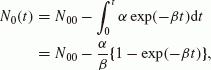(1)

where, N00 is the initial number N0(0), i.e., 20,062 in the present case.

The values of α and β are easily obtained if we assume that observation and the fitted curve coincide with each other at the middle and the last points, i.e., t = 22 and 44 years, respectively. Since N0(22) = 18,963 and N0(44) = 18,150 in the observation, Eq. (1) is rewritten as follows assuming x = exp(−22β):(2)

Solutions of this equation, i.e., x 0.740 and just 1, result in α 57.9 and β 0.0137. Another result obtained by least-square fitting is also close to these values, i.e., α 58.3 and β 0.0143. As shown in Figs. 2(a) and 2(b), the fitted curves describe well the observation. The difference between the values of α and β obtained by different methods is difficult to discriminate in the figure.

The values of α and β indicate that N0(t) does not converge to zero but to N00α/β, i.e., roughly about 16,000 in the present case, as t → ∞. In other words, for about 80% of the whole area, earthquakes with a size considered in the present discussions, i.e., M 5, are not expected to occur. Since this is a rough estimation based only on the recent 44 years, detailed discussions are left for further considerations.

In the discussion above, the probability p0(t)dt that an earthquake will occur in a cell of zero activity is not yet considered directly. A definition of p0(t) is expressed by the following equation: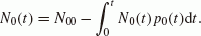(3)

Based on Eqs. (1) and (3), p0(t)dt can be written as follows: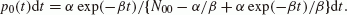(4)

This shows that p0(t) decreases to zero as t →∞ .However, it is somewhat questionable that a temporal change of the probability occurs at each cell. It is more likely that each cell has its own constant rate of earthquake occurrence. Then earthquakes are likely to occur more often at the cells with a higher rate of activity. Consequently, the cells with a smaller rate will be left behind, and their average rate will become smaller and smaller as shown in Eq. (4).

The expected number of earthquakes at a cell of zero activity, i.e., λ0(t)dt, is expressed as follows using a wellknown relation between probability and expected number: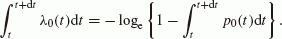(5)

For example, the values for t = 0.5 and 43.5 years (i.e., the values at the middle of 1965 and 2008, respectively) are P0(0.5) = 0.0029, λ0(0.5) = 0.0029, p0(43.5) = 0.0017 and λ0(43.5) = 0.0017, respectively. The values of p0(t) and λ0(t) are almost equal to each other as long as they are small.

## 3. Cells with Previous Activity

In the discussion above, a cell of zero activity has been considered. In this section, the activity at a cell in which some earthquakes have previously occurred is formulated, and then the temporal change in the distribution N n is verified by a simulation. Here, N n is the number of cells in which n earthquakes have occurred in previous years.

At a cell in which n earthquakes have occurred in the arbitrary determined period, i.e., a reference period Tr, assume λ n to be the expected number of earthquakes that will occur in the forecast period T. The value of λ n may be considered to be the product of an average activity per unit time (n/Tr) and the duration of the forecast period (T). However, this is not correct because a certain number of earthquakes are expected to occur in the forecast period at places where no earthquakes have occurred before. For example, at a cell where earthquakes have occurred once per year on average in previous years, the expected number should not be just 1 in the next year.

In order to adjust the total number of earthquakes to coincide with the long-term average over the whole area, λn is assumed to be as follows:(6)

where n is equal to or larger than 1;Y is a long-term average of the total number of earthquakes during the period T (the yearly average is obtained as 78.05 based on the data from 1965 to 2008); N0 is the number of cells of zero activity just before the forecast period; λ0 is the expected number of earthquakes that occur at a cell of zero activity. N0 and λ0 are originally functions of time. For simplicity, however, they are assumed here to be a constant through the respective forecast period. That is, if t is the time of the beginning of a forecast period, then N0 = N0(t) and λ0 is an integral of λ0(t)dt from t to t + T. When the temporal change of λ0(t) is not so large in the period T, λ0 may be approximated by T λ0(t + T/2).

Based on Eqs. (5) and (6), a forecast becomes possible at all cells. In order to justify the present formulation, taking 1 year for the forecast period, the temporal change in the distribution N n , i.e., the number of cells with n earthquakes in the reference period, is simulated year by year as follows.

When the expected number is λ, the probabilities that 0, 1, 2 and m earthquakes will occur, i.e., P0, P1, P2, and P m , respectively, are expressed by the following well-known relations: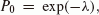(7)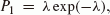(8)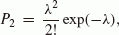(9)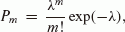(10)

where λ is λ0 or λ n according to the previous activity.

In the simulation, for each of the 20,062 cells, a random number R between 0 and 1 is generated. If R < P0, it is regarded that no earthquakes occur at this time step. Otherwise, if P0< R < P0+ P1 or P0 + P1< R < P0+ P1+ P2, for example, then it is regarded that one or two earthquakes occur, respectively. In that case, if λ = λ n , N n in the next step should be decreased by 1, and N n +1 or N n +2 in the next step is increased by 1 according to the value of R. Repeating this process 100 times, the average distribution of N n after 22 and 44 steps are shown in Table 1, with observations at the end of 1986 and 2008 starting from the beginning of 1965.

The results suggest a good correlation between simulation and observation. The coincidence of N0 is not particularly significant because the parameters in p0(t) and, thus, the value of λ0 in this section are obtained to fit the temporal change in N0 itself. However, the fact that the values of N1, N2, N3, and others, also represent a good correlation can be regarded as evidence for the justification of Eq. (6), at least to some extent. Contrary to the assumption in Eq. (6), an alternative hypothesis may be that the place at which earthquakes have occurred before will be a location of low activity. Such a possibility is, however, denied implicitly by the present simulation.

As shown in Table 1, there are several cells at which the activity was strongly concentrated. Based on the relative intensity model, it will not be easy to reproduce such concentrations. However, it is still interesting that high values between 21 and 27 appear 14 times among the 100-trial simulation of 44 steps. Although the occurrence rate does not attain the observed value, a concentration of activity itself may occur in some cases.

## 4. Spatial Correlation of Long-term Activity

In the previous sections, the long-term average of seismic activity is discussed independently at each unit cell of size 0.1° by 0.1° in latitude and longitude. It is, however, probably incorrect to assume that there are no correlations between neighboring or near-by cells with a size of only 0.1°. In order to determine an optimal size of the reference area, various distances in latitude and longitude are compared such as 0.1°, 0.2°, and 0.3°. Here, the target cell is located in the center of the reference area. For example, when the size of the reference area is 0.3°, it is taken to be ±0.15° in latitude and longitude around the center of the target cell.

A statistical consideration is carried out as follows. For earthquakes with a magnitude of 5 or greater and a depth of 100 km or less, a long-term average of activity is obtained at each cell for the period of 43 years from 1965 to 2007, and for the assumed size of the reference area. According to this long-term average, the expected number of earthquakes for 2008 is forecast at each cell using the value of λ0 or λ n .

In the case that the reference area is not confined to a unit cell, it is not straightforward to estimate λ0. Consequently, various values of λ0 are assumed to find the best model. In the present case, the expected number, λ n , is also not the value described by Eq. (6) but,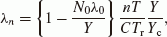(11)

where,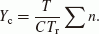(12)

Here, Yc is the long-term average of the total number of earthquakes per unit cell and time period T, smoothed over the reference area characterized by the parameter C ; C is the ratio of the reference area to the area of a unit cell; n is re-determined to be the observed numberin the reference area during the reference period Tr; Y equals to Y1 and is the same as in Eq. (6). According to this correction, the total number of earthquakes forecasted, i.e., the summation of λ0 and λn over the whole area, corresponds to Y.

This retrospective forecast is then compared with observation in terms of the log-likelihood. The values of likelihood (L) and log-likelihood (logeL) are: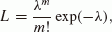(13)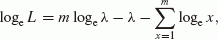(14)

respectively. Here, λ and m are the forecast and observation, respectively. The likelihood test is not the only tool to evaluate the performance of forecast models, but various methods are applied or prepared in the CSEP testing centers (e.g., Schorlemmer et al., 2010; Zechar et al., 2010b). In the present paper, however, the value of likelihood is used to find a starting model for advanced discussions, because of its convenience.

Similarly, 1-year forecasts from 1989 to 2007 are carried out based on the data of the previous 43 years. Results of the experiments are shown in Figs. 3(a) and 3(b) by black curves for various reference areas. In both figures, the vertical axis represents the total log-likelihood of 1-year forecasts from 1989 to 2008. The horizontal axes in Figs. 3(a) and 3(b) are the assumed expected value λ0 and s0, that is λ0 multiplied by the factors of C and Tr, i.e., s0= λ0CTr. Here, the reference period Tr is 43 years in the present case. The value s0 in Fig. 3(b) may be convenient for comparison with a value at a cell in which only 1 earthquake has occurred in the reference period.

As shown in Figs. 3(a) and 3(b), the maximum value is obtained when the reference area is 0.3° in latitude and longitude and λ0 or s0 is around 0.00085 per year or 0.33, respectively. The cases of 0.2° and 0.4° are the next best, i.e., the maximum values of the total log-likelihood are less than that of 0.3° by about 10 and 41, respectively. In the case of 0.1°, the maximum value of the total log-likelihood is far less than that of 0.3°. Since the difference is more than 390, 0.1° is not an appropriate value in a plausible model for a forecast. When the reference area extends to 0.5° or greater in size, the total log-likelihood decreases markedly.

Incidentally, in the case of 0.1°, the maximum value of the total log-likelihood is obtained when λ0 is about 0.0019. This result almost coincides with the value obtained previously using Eqs. (4) and (5), although the reference periods are not equal to each other. That is, in the present figures, the reference period goes back to 1946, for example, in the case of the forecast for 1989.

## 5. Exclusion of the Previous Year

In the relative intensity model, a temporal relation between the main shock and aftershocks is partly included. That is, if a large earthquake occurs at the end of the reference period and aftershocks continue into the subsequent forecast period, then a long-term average that is generally higher at the focal region contributes to the performance of the forecast. In the case of a fore- and main-shock sequence, an earthquake swarm or any other kind of correlated earthquakes that occur both in the reference and the forecast periods, leads to the same situation. Such correlations, if any, may appear more clearly when the reference area is taken to be small. On the contrary, when the reference area is taken to be very large, any correlation will be diluted by areal averaging.

Taking this into consideration, it will be necessary to examine whether the relation between the extent of the reference area and the value of the log-likelihood may change as a result of the effects of correlated earthquakes. If the year just before the forecast period is excluded from the reference period, such an effect will be drastically decreased. Accordingly, the 1-year forecast for 2008, for example, is recalculated based on the average of 43 years from 1964 to 2006, shifted backward by 1 year.

The total values of the re-calculated log-likelihood for the 20 years from 1989 to 2008 are superposed by gray curves in Figs. 3(a) and 3(b). Although the curves are all lower than the corresponding black curves, this conclusion that the maximum value is obtained for a reference area of 0.3° in size is unaffected. In order to intensify these relations, the maximum values of the log-likelihood of the respective black and gray curves are replotted in Fig. 4 as a function of the size of the reference area. The difference between the black and gray curves, i.e., the difference with, or without, the year just before the forecast period, is 91, 58, 65, 52, and 50 on the log scale for a reference area with a size of 0.1°, 0.2°, 0.3°, 0.4°, and 0.5°, respectively.

## 6. Duration of the Reference Period

In general, the long-term average will be more reliable when the reference period is long. In contrast, a possible effect due to correlated activity, such as aftershocks, is likely to appear when the reference period is short. Consequently, the difference in log-likelihood is compared for various durations of the reference period.

Similar to the previous discussions, the maximum total log-likelihood of 1-year forecasts from 1989 to 2008 is shown by squares in Fig. 5. The log-likelihood increases rapidly up to around 40–43 years for the reference period. Beyond this period, any improvement in the log-likelihood is not clear. Therefore, there is no reason to extend the reference period to be more than around 40–43 years. In Sections 4 and 5, the results are discussed with a reference period of 43 years, which is shown to be nearly the best duration of the reference period.

In the present investigation, 1-year forecasts from 1989 to 2008 are carried out retrospectively. This means that data in the 1920s to 1940s are considered if the duration of the reference period exceeds 40 years. As for the earthquakes with a magnitude of 5 or greater, incompleteness of the earthquake catalog may not be so serious even in these earlier periods. However, their locations could have poor accuracy. This may be the reason why the log-likelihood does not improve when the reference period increases to 50–70 years.

Referring only to recent 1-year forecasts, however, the best log-likelihood seems to be obtained when the reference period is as long as possible. For example, the total log-likelihood of 1-year forecasts of the 5 years from 2004 to 2008 increases gradually up to an upper limit of around 80 years of the reference period, as shown by circles in Fig. 5. Because of the lack of a sufficient duration of the available period, detailed discussions are left for further investigations.

## 7. Conclusions

An improvement in earthquake forecasts using the long-term average of previous seismic activity, i.e., the relative intensity model, is sought in the Japan area for earthquakes with a magnitude of 5 or greater and a depth of 100 km or less. The area is divided into 20,062 cells with a size of 0.1° in latitude and longitude. Among various combinations of parameters, the best log-likelihood is obtained when (1) the expected number of earthquake occurrences at a cell of zero activity is estimated to be 0.00085 per year for a reference period of 43 years, and (2) the reference area is 0.3° by 0.3° in latitude and longitude. Different values of these parameters result in poorer values for the log-likelihood.

In addition, (3) the best duration of the reference period is tentatively found to be around 40–43 years. However, there may be an alternative possibility that the performance of the forecasts in the latest years is improved when the reference period is as long as possible, i.e., around 80 years in the present case. As more earthquake data is accumulated, further discussions of this aspect will be desirable in the future.

The size of 0.3° of the reference area may partly reflect the accuracy of hypocentral determination by the Japan Meteorological Agency. In the present case, of 9 unit cells in a reference area of 0.3° in size, the activity of the central unit cell correlates only with the surrounding 8 cells. In other words, the correlation becomes poor when the distance from the center of the unit cell exceeds 0.15° in latitude or longitude.

The optimal values discussed in the present paper may change for different areas in the world. Therefore, a comparison of the differences, or similarities, between respective areas is interesting to investigate, although the accuracy of data in the available earthquake catalog may also affect the results. Since the long-term average of earthquake occurrences is a fundamental basis for forecasts, the present values themselves, or discussions on how the values are obtained, may help to improve forecast models in various areas. Although the relative intensity model is almost time-independent, only to forecast the background activity, an appropriately-optimized relative intensity model may be expected to show an improved performance in combination with time-dependent forecast models. In the present paper, only log-likelihoods have been compared to evaluate the performance of the forecast. Detailed discussions with additional tests are also left for further studies.

## References

• Allen, C. R., P. S. Amand, C. F. Richter, and J. M. Nordquist, Relationship between seismicity and geologic structure in the southern California region, Bull. Seismol. Soc. Am., 55, 753–797, 1965.

• Gutenberg, B. and C. F. Richter, Frequency of earthquakes in California, Bull. Seismol. Soc. Am., 34, 185–188, 1944.

• Gutenberg, B. and C. F. Richter, Seismicity of the Earth and Associated Phenomena, Princeton Univ. Press, 1–273, 1949.

• Helmstetter, A., Y. Y. Kagan, and D. D. Jackson, High-resolution time-independent grid-based forecast for M ≥ 5 earthquakes in California, Seismol. Res. Lett., 78, 78–86, 2007.

• Holliday, J. R., K. Z. Nanjo, K. F. Tiampo, J. B. Rundle, and D. L. Turcotte, Earthquake forecasting and its verification, Nonlin. Proc. Geophys., 12, 965–977, 2005.

• Holliday, J. R., C. C. Chen, K. F. Tiampo, J. B. Rundle, D. L. Turcotte, and A. Donnellan, A RELM earthquake forecast based on pattern informatics, Seismol. Res. Lett., 78, 87–93, 2007.

• Ishikawa, Y., Change of JMA hypocenter data and some problems, Quart. J. Seismol., 51, 47–56, 1987 (in Japanese with English abstract).

• Jordan, T., Earthquake predictability, brick by brick, Seismol. Res. Lett., 77, 3–6, 2006.

• Kafka, A. L., Statistical analysis of the hypothesis that seismicity delineates areas where future large earthquakes are likely to occur in the central and eastern United States, Seismol. Res. Lett., 73, 992–1003, 2002.

• Kagan, Y. Y. and D. D. Jackson, Probabilistic forecasting of earthquakes, Geophys. J. Int., 143, 438–453, 2000.

• Kagan, Y. Y., D. D. Jackson, and Y. F. Rong, A testable five-year forecast of moderate and large earthquakes in southern California based on smoothed seismicity, Seismol. Res. Lett., 78, 94–98, 2007.

• Kossobokov, V. G., Earthquake prediction: Basics, achievements, perspectives, Acta Geod. Geophys. Hung., 39, 205–221, 2004.

• Mallet, R., Fourth report upon the facts and theory of earthquake phenomena, Rep. British Assoc. Adv. Sci., 1–136, 1858.

• Nanjo, K. Z., Earthquake forecasts for the CSEP Japan experiment based on the RI algorithm, Earth Planets Space, 63, 261–274, 2011.

• Nanjo, K. Z., J. B. Rundle, J. R. Holliday, and D. L. Turcotte, Pattern informatics and its application for optimal forecasting of large earthquakes in Japan, Pure Appl. Geophys., 163, 2417–2432, 2006.

• Nanjo, K. Z., H. Tsuruoka, N. Hirata, and T. H. Jordan, Overview of the first earthquake forecast testing experiment in Japan, Earth Planets Space, 63, 159–169, 2011.

• Nishenko, S. P. and G. A. Bollinger, Forecasting damaging earthquakes in the central and eastern United States, Science, 249, 1412–1416, 1990.

• Rhoades, D. A. and F. F. Evison, Long-range earthquake forecasting with every earthquake a precursor according to scale, Pure Appl Geophys., 161, 47–72, 2004.

• Rundle, J. B., K. F. Tiampo, W. Klein, and J. S. Sa Martins, Self-organization in leaky threshold systems: the influence of near-mean field dynamics and its implications for earthquakes, neurobiology, and forecasting, Proc. Natl. Acad. Sci. USA, 99, 2514–2521, 2002.

• Schorlemmer, D., J. D. Zechar, M. J. Werner, E. H. Field, D. D. Jackson, T. H. Jordan, and RELM Working Group, First results of the regional earthquake likelihood models experiment, Pure Appl. Geophys., 167, 859–876, doi:10.1007/s00024-010-0081-5, 2010.

• Sekiya, S., Large earthquakes in Japan, Rep. Earthq. Inv. Comm., 26, 1–8, 1899 (in Japanese).

• Tiampo, K. F., J. B. Rundle, S. McGinnis, S. J. Gross, and W. Klein, Mean-field threshold systems and phase dynamics: An application to earthquake fault systems, Europhys. Lett., 60, 481–487, 2002.

• Tsuruoka, H., N. Hirata, D. Schorlemmer, F. Euchner, K. Z. Nanjo, and T. H. Jordan, CSEP Testing Center and the first results of the earthquake forecast testing experiment in Japan, Earth Planets Space, 64, this issue, 661–671, 2012.

• Wessel, P. and W. H. F. Smith, New, improved version of Generic Mapping Tools released, Eos Trans. AGU, 79, 579, 1998.

• Zechar, J. D. and T. H. Jordan, Simple smoothed seismicity earthquake forecasts for Italy, Ann. Geophys., 53 (3), 99–105, doi:10.4401/ag-4845, 2010.

• Zechar, J. D., D. Schorlemmer, M. Liukis, J. Yu, F. Euchner, P. J. Maechling, and T. H. Jordan, The Collaboratory for the Study of Earthquake Predictability perspective on computational earthquake science, Concurrency Computat. Pract. Exper., 22, 1836–1847, doi:10.1002/cpe.1519, 2010a.

• Zechar, J. D., M. C. Gerstenberger, and D. A. Rhoades, Likelihood-based tests for evaluating space-rate-magnitude earthquake forecasts, Bull. Seismol. Soc. Am, 100, 1184–1195, doi:10.1785/0120090192, 2010b.

## Acknowledgements

The authors would like to express their gratitude for helpful comments and suggestions of Professors James J. Mori (Kyoto Univ.), John B. Rundle (Univ. Calif. Davis), and Rodolfo Console (Ist. Naz. Geofis. Vulcanol.), and Drs. J. Douglas Zechar (ETH Zurich, Swiss Seism. Service), Tetsuto Himeno (Nat. Inst. Polar Res.), Takeo Ishibe, and Hiroshi Tsuruoka (Univ. Tokyo). The authors are also grateful to the staffs of the Japan Meteorological Agency and related organizations for their continuing efforts of seismic observation and data analysis. Figure 1 is drawn using the Generic Mapping Tools (GMT) of Wessel and Smith (1998).

## Author information

Authors

### Corresponding author

Correspondence to Ken’ichiro Yamashina.

## Rights and permissions

The images or other third party material in this article are included in the article’s Creative Commons licence, unless indicated otherwise in a credit line to the material. If material is not included in the article’s Creative Commons licence and your intended use is not permitted by statutory regulation or exceeds the permitted use, you will need to obtain permission directly from the copyright holder.

To view a copy of this licence, visit https://creativecommons.org/licenses/by/4.0/.

Reprints and Permissions

Yamashina, K., Nanjo, K.Z. An improved relative intensity model for earthquake forecasts in Japan. Earth Planet Sp 64, 683–691 (2012). https://doi.org/10.5047/eps.2011.02.002

• Revised:

• Accepted:

• Published:

• Issue Date:

• DOI: https://doi.org/10.5047/eps.2011.02.002

### Key words

• Earthquake forecast
• CSEP
• long-term average
• relative intensity
• zero activity